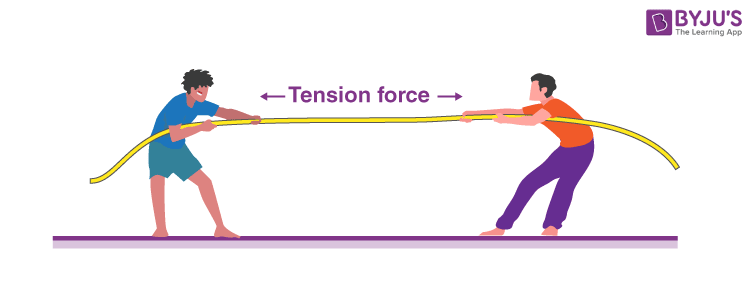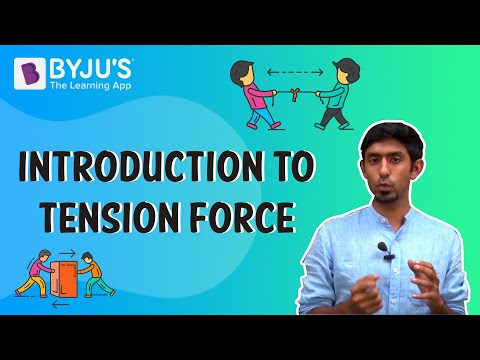# Tension ForceTension is defined as the force transmitted through a rope, string or wire when pulled by forces acting from opposite sides. The tension force is directed over the length of the wire and pulls energy equally on the bodies at the ends.

# What is Tension Force?

Every physical object which is in contact exerts some force on one another. These contact forces are assigned names based on the kind of objects. If one of the forces exerting an object is a rope, cable or chain, you can call it tension.

Cables and Ropes can be used for exerting forces since they can efficiently transfer a force over a specific distance (e.g. the rope length). Please note that tension is the pulling force since ropes cannot push effectively. Pushing with a rope causes the rope to go slack and lose the tension that allowed it to pull in the original place. This might seem obvious, but when it is about drawing the forces acting on an object, some often draw the force of tension going in the wrong direction. Hence, tension can only pull an object.

In almost every situation in classical mechanics, you will be presented with massless ropes and cables. When a rope is massless, it transfers the force from one end to the other perfectly.

For instance, if a person pulls a massless rope with a force of 20 N, the block also experiences a force of 20 N. All massless ropes experience two opposite and equal tension forces. Here, the person pulling a block with a rope, rope experiences a net force. Therefore, all massless ropes experience two opposite and equal tension forces. In the case of a person pulling a block, the rope experiences tension in one direction from the pull and tension in another direction from the reactive force of the block.

## Tension Force Formula

The tension in a body can be expressed numerically as follows:

T = mg + ma

Where;

T indicates tension, N

m indicates mass, kg

g indicates gravitational force, 9.8 m/s2

A indicates acceleration, m/s2## Frequently Asked Questions – FAQs

Q1

### What is tension in force?

In physics, a tension force is a force that develops in a rope, thread, or cable as it is stretched under an applied force.
Q2

### What is the source of the tension?

When atoms or molecules are driven away from one other and accumulate potential energy while a restoring force remains, the restoring force may generate what is also known as tension.
Q3

### Is tension and elastic force?

Tension is a pulling force connected with an item such as a rope, cable, or chain. It is analogous to elastic potential energy.
Q4

### Why tension is a electromagnetic force?

Tension is caused by the interactions between the molecules that comprise the thread, spring, or whatever. The electromagnetic force is actually everything that exists.
Q5

### Which type of force is tension in a string?

Electromagnetic force.
Test Your Knowledge On Tension Force!